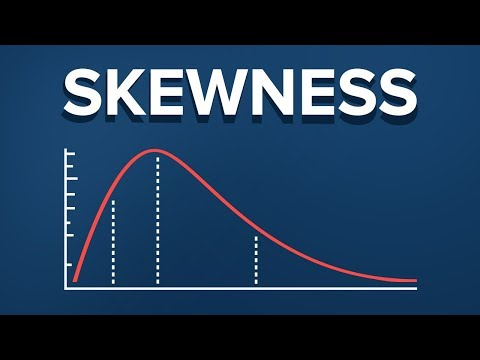Skewness Example

An Example of Skewness

The most commonly used tool to measure asymmetry is skewness.

This is the formula to calculate it. Almost always, you will use software that performs the calculation for you, so in this lesson, we will not get into the computation, but rather the meaning of skewness.

So, skewness indicates whether the observations in a data set are concentrated on one side.

Skewness can be confusing at the beginning, so a skewness example is in place.

Remember frequency distribution tables from previous lectures? Here we have three data sets and their respective frequency distributions. We have also calculated the means, medians and modes.

The first data set has a mean of 2.79 and a median of 2, hence the mean is bigger than the median. We say that this is a positive or right skew. From the graph, you can clearly see that the data points are concentrated on the left side. Note that the direction of the skew is counterintuitive. It does not depend on which side the line is leaning to, but rather to which side its tail is leaning to. So, right skewness means that the outliers are to the right.

It is interesting to see the measures of central tendency incorporated in the graph.

When we have right skewness, the mean is bigger than the median, and the mode is the value with the highest visual representation.

In the second graph, we have plotted a data set that has an equal mean, median and mode. The frequency of occurrence is completely symmetrical and we call this a zero or no skew. Most often, you will hear people say that the distribution is symmetrical.

For the third data set, we have a mean of 4.9, a median of 5 and a mode of 6. As the mean is lower than the median, we say that there is a negative or left skew. Once again, the highest point is defined by the mode. Why is it called a left skew, again? That’s right, because the outliers are to the left.

Alright. So, why is skewness important? Skewness tells us a lot about where the data is situated. As we mentioned in our previous lesson, the mean, median and mode should be used together to get a good understanding of the dataset. Measures of asymmetry like skewness are the link between central tendency measures and probability theory, which ultimately allows us to get a more complete understanding of the data we are working with.

Hope that our skewness example came in handy. Curious to learn more? Jump to our statistics tutorials or check out our all-in-one Data Science Training.Data Science PR

This site uses Akismet to reduce spam. Learn how your comment data is processed.

Recent posts

Hedera Hashgraph Price Analysis 2021 – HBAR Token Price Forecast 2021 -Hbar Price Prediction 2021Don't be shy, get in touch. We love meeting interesting people and making new friends.